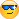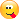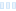Hi All,
I'm working on a new gameThe formula is TMan in Russell Walsmith orgform.
Basically is my SlopeTMan that i'm rewritng and re-structured but i have a problem.
I have implemented the TMan of Russell and i added also a normal Mandelbrot that i attach under.
In your opinion is it correct?
That parameter is correct?
Try it to p1 (0.0 , 0.1), you will see the black line, i think is missing of something but i don't understand what.

The set is correct

``````  x2 = x * x - y * y - z * z + cx;
y2 = 2 * x * y + cy;
z2 = 2 * x * z + cz;
``````

This is the formula

``````
TMan{
c1=real(pixel)
c2=imag(pixel)
c3=p1
z1=z2=z3=0

:

t1=z1^2-z2^2-z3^2
t2=2*z1*z2
t3=2*z1*z3
z1=t1+c1
z2=t2+c2
z3=t3+c3

z=z1+z2+z3
|z| < 64
}
``````
Hi All, I&#039;m working on a new game 8) The formula is TMan in Russell Walsmith orgform. Basically is my SlopeTMan that i&#039;m rewritng and re-structured but i have a problem. I have implemented the TMan of Russell and i added also a normal Mandelbrot that i attach under. In your opinion is it correct? That parameter is correct? Try it to p1 (0.0 , 0.1), you will see the black line, i think is missing of something but i don&#039;t understand what. The set is correct x2 = x * x - y * y - z * z + cx; y2 = 2 * x * y + cy; z2 = 2 * x * z + cz; This is the formula ```` TMan{ c1=real(pixel) c2=imag(pixel) c3=p1 z1=z2=z3=0 : t1=z1^2-z2^2-z3^2 t2=2*z1*z2 t3=2*z1*z3 z1=t1+c1 z2=t2+c2 z3=t3+c3 z=z1+z2+z3 |z| &lt; 64 } ````

Andrea Spinozzi

https://fractalcosmo.com
edited 5 days ago at 5:38 pm

0

At the end i don't find any solution, or better i can change planes using c3 but the corret loop i think is this.
Some suggests are appreciate, the question is PARAMETER 1 is working correctly? I think yes.
Suggests?

``````TMan{
z1=z2=z3=0
c1=real(pixel)
c2=imag(pixel)
c3=p1

:

t1= z1^2-z2^2-z3^2
t2= 2*z1*z2
t3= 2*z1*z3

z1=t1+c1
z2=t2+c2
z3=t3+c3
z=z1^2+z2^2+z3^2

|z| < 64
}
``````
At the end i don&#039;t find any solution, or better i can change planes using c3 but the corret loop i think is this. Some suggests are appreciate, the question is PARAMETER 1 is working correctly? I think yes. Suggests? ```` TMan{ z1=z2=z3=0 c1=real(pixel) c2=imag(pixel) c3=p1 : t1= z1^2-z2^2-z3^2 t2= 2*z1*z2 t3= 2*z1*z3 z1=t1+c1 z2=t2+c2 z3=t3+c3 z=z1^2+z2^2+z3^2 |z| &lt; 64 } ````

Andrea Spinozzi

https://fractalcosmo.com

0

Hi, i understood the problem, because basically that set is a 3D Quat, the parameter 1 work correctly in the real part and in a normal Quat, the real and image part of cj ck are the same, you can see it in fractint colletion, quaternion mandelbrot, instead in this version the real part is ok but the image part is creating that black line but only in p1=(0.0,0.1) so using image part at 0.1, or 0.2,0.3 using the image part, the solution can be add a low value at zk and UF does the iteration and the black line disappears.
BTW next days i'll upload the formula on the DB so you can see it, SlopeTMan, i wanted upload it here before that in the UFDB but i think i can't because i tried yesterday but the formula is 2240 lines and i think exceeds the maximum lines allowed in a post, because is trunked.
Ciao a tutti e buon week-end

``````TMan{
z1=z2=z3=0.0
pk=1e-3
c1=real(#pixel)
c2=imag(#pixel)
c3=p1

:

t1= z1^2-z2^2-z3^2
t2= 2*z1*z2
t3= 2*z1*z3+pk

z1=t1 + c1
z2=t2 + c2
z3=t3 + c3
z=z1^2+z2^2+z3^2

|z| < 128
}
``````
Hi, i understood the problem, because basically that set is a 3D Quat, the parameter 1 work correctly in the real part and in a normal Quat, the real and image part of cj ck are the same, you can see it in fractint colletion, quaternion mandelbrot, instead in this version the real part is ok but the image part is creating that black line but only in p1=(0.0,0.1) so using image part at 0.1, or 0.2,0.3 using the image part, the solution can be add a low value at zk and UF does the iteration and the black line disappears. BTW next days i&#039;ll upload the formula on the DB so you can see it, SlopeTMan, i wanted upload it here before that in the UFDB but i think i can&#039;t because i tried yesterday but the formula is 2240 lines and i think exceeds the maximum lines allowed in a post, because is trunked. Ciao a tutti e buon week-end ```` TMan{ z1=z2=z3=0.0 pk=1e-3 c1=real(#pixel) c2=imag(#pixel) c3=p1 : t1= z1^2-z2^2-z3^2 t2= 2*z1*z2 t3= 2*z1*z3+pk z1=t1 + c1 z2=t2 + c2 z3=t3 + c3 z=z1^2+z2^2+z3^2 |z| &lt; 128 } ````

Andrea Spinozzi

https://fractalcosmo.com

0

Resolved....
This is a solution, but yes there is nothing of wrong, just decide how parameter 1 must work or better, image part of parameter 1.
This is a solution but i don't know if i will use this solution or the solution added a low value to p1, because in my formula there are also other combinations, there are 4 hyper complex parameters, this is just one. But there is nothing of wrong.
BTW this is a solution, p1 now works correctly like as Quat Mandelbrot in fractint collection.

``````TMan-C{
zr=0
zi=0
zk=0
c1=real(#pixel)
c2=imag(#pixel)
c3=p1

:

zkr=real(zk)
zki=imag(zk)
t1= zr^2 - zi^2 - zkr^2 - zki^2
t2= 2*zr*zi
t3= 2*zr*zkr + flip(2*zr*zki)

zr=t1 + c1
zi=t2 + c2
zk=t3 + c3
z=zr+flip(zi)

|z|+|zk| < 128
}
``````
Resolved.... This is a solution, but yes there is nothing of wrong, just decide how parameter 1 must work or better, image part of parameter 1. This is a solution but i don&#039;t know if i will use this solution or the solution added a low value to p1, because in my formula there are also other combinations, there are 4 hyper complex parameters, this is just one. But there is nothing of wrong. BTW this is a solution, p1 now works correctly like as Quat Mandelbrot in fractint collection. ```` TMan-C{ zr=0 zi=0 zk=0 c1=real(#pixel) c2=imag(#pixel) c3=p1 : zkr=real(zk) zki=imag(zk) t1= zr^2 - zi^2 - zkr^2 - zki^2 t2= 2*zr*zi t3= 2*zr*zkr + flip(2*zr*zki) zr=t1 + c1 zi=t2 + c2 zk=t3 + c3 z=zr+flip(zi) |z|+|zk| &lt; 128 } ````

Andrea Spinozzi

https://fractalcosmo.com

0

No guys nothing done, I have decided that in the new SlopeTMan I will not put the normal Mandelbrot Set because I don't like how it works, in the formula there are also the variations of the planes the 3D dimensions and if the Triternion is not composed correctly, changing the planes , hyper complex parameters behave incorrectly. Using Russell's TMan the structure is correct and all the parameters work correctly if I try to insert a normal Mandebrot it does not work as it should so the setting is wrong, in fact I was just exploring possible sets.No guys nothing done, I have decided that in the new SlopeTMan I will not put the normal Mandelbrot Set because I don&#039;t like how it works, in the formula there are also the variations of the planes the 3D dimensions and if the Triternion is not composed correctly, changing the planes , hyper complex parameters behave incorrectly. Using Russell&#039;s TMan the structure is correct and all the parameters work correctly if I try to insert a normal Mandebrot it does not work as it should so the setting is wrong, in fact I was just exploring possible sets. 8) :P

Andrea Spinozzi

https://fractalcosmo.com
edited 2 days ago at 7:49 pm

0

Hi,
At the end i must share the formula, i attach the link where you find as2.ufm + SlopeTMan, i renamed in asNew for comfort when uploading from UF, here there is SlopeTMan.
There is for sure the Triternion Mandelbrot changing C , but i wanted add also a normal Mandelbrot but with this structure is not so simple.
The formula is good and the 3R dimension are very usefull.
Ciao a tutti

https://fractalcosmo.com/as2New.zip

Hi, At the end i must share the formula, i attach the link where you find as2.ufm + SlopeTMan, i renamed in asNew for comfort when uploading from UF, here there is SlopeTMan. There is for sure the Triternion Mandelbrot changing C , but i wanted add also a normal Mandelbrot but with this structure is not so simple. The formula is good and the 3R dimension are very usefull. Ciao a tutti https://fractalcosmo.com/as2New.zip

Andrea Spinozzi

https://fractalcosmo.com

0
49
views
5
replies
1
followers
live preview
Enter at least 10 characters.
WARNING: You mentioned %MENTIONS%, but they cannot see this message and will not be notifiedSaving...
Saved
All posts under this topic will be deleted ?
Pending draft ... Click to resume editing